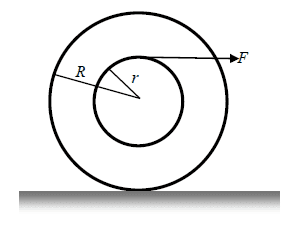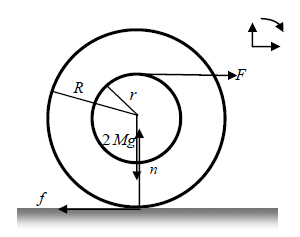# In which direction does the friction on the wheel go?

## Homework StatementThe following wheel consists of an outer ring (R) and an inner ring (r). The wheel moves clockwise by a force F applied to the inner ring as shown in the picture to make the wheel roll.
Draw the friction force.

Nothing

## The Attempt at a Solution

I could have sworn that if the wheel is turning clockwise as it moves, then the friction between it and the ground is drawn to the right because as it moves clockwise, the friction force would be directed counter-clockwise.

However my facit shows that the friction force is to the left:http://[URL]http://i.imgur.com/l2ZjUNO.png [Broken]
Isn't this wrong? Shouldn't the friction force be drawn in the opposite direction? Or am I misunderstanding something here? (ignore the gravity and normal force in the drawing)

Last edited by a moderator:

jedishrfu
Mentor
•PhyIsOhSoHard
The applied force F is causing the wheel to move to the right so the friction force f opposes that hence it points to the left.

Maybe this explanation will help:

http://www.phy.davidson.edu/fachome/dmb/PY430/Friction/rolling.html

Oh I see. If the situation was where the wheel was standing at rest and didn't move then the friction force would be to the right to prevent it from rolling but since the wheel is rolling that means our friction force is to the left, correct?

jedishrfu
Mentor
That seems right.

•PhyIsOhSoHard
Stephen Tashi
If "the friction force" means the force exerted by the wheel on the horizontal surface then it points one way. If "the friction force" means the force exerted by the surface on the wheel, then it points in the opposite direction.

Let's talk about the force exerted by the surface on the wheel. Thinking intutively, imagine what would happen without much friction. Would the wheel tend to "roll faster than it slides" or "slide faster than it rolls"? (i.e. is the tangential speed of a point on the perimeter of the wheel greater or less than the linear speed of the wheel.) My bias is toward visualizing "roll faster than it slides" due to familiarity with automobile wheels. However, I think you could have "slides faster than it rolls" situation if we imagine radius $r$ to be very small so the force $F$ is acting almost at the center of the wheel.

In analyzing problems, you can draw vectors in the wrong direction and still do a valid analysis if you interpret a negative magnitude as telling you that the direction of the vector is opposite to the way you drew it.

•PhyIsOhSoHard
haruspex